# Use VLOOKUP from Two or More Lookup Tables

Imagine, you have two tables of students. You want to do a lookup for Kyle in table1. If he’s not found in table1, you would want to search in the other table.
Or you if you want to switch lookup tables based on some conditions.
In both cases, you have to look up more than one lookup tables. So how do you use VLOOKUP from two Lookup tables or more. This article solves this problem very easily.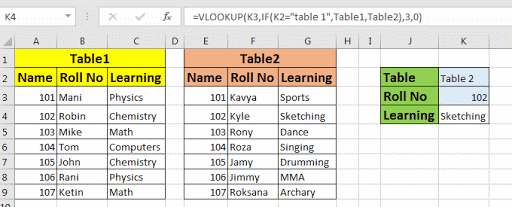Let’s solve above two VLOOKUP problems and understand how you can switch lookup tables.
Change Lookup Table If Lookup Value Is Not Found In First Lookup Table.
Here I have two tables that contains data of students in class1 and class2. I want to lookup for “kyle” in table1 and if not found, I want do VLOOKUP in second table.

I have named first table as Class1 (A3:C9) and second table as Class2 (E3:G9).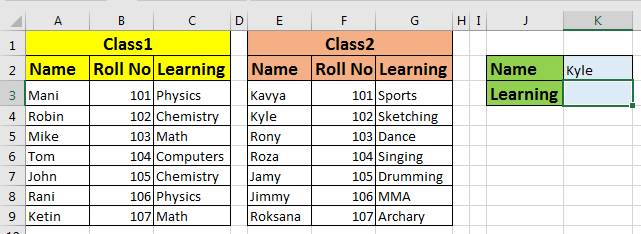Here we will use IFERROR function to trap error and change the lookup range.

=IFERROR(VLOOKUP(K2,Class1,3,0),VLOOKUP(K2,Class2,3,0))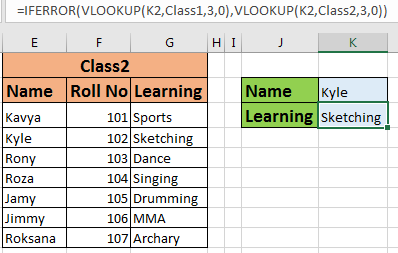How It Works

IFERROR checks if VLOOKUP function is returning error or not, if it returns any error, it returns value_if_error part. In that part, we have another VLOOKUP function that looks up in the different lookup array. It is simple. If you have to lookup in more than 2 tables then nest each IFERROR function with another IFERROR function. This will make a chained VLOOKUP function that will lookup in different tables.

Change Lookup Array Based on a Condition
In above case we wanted to switch VLOOKUP formula, if first one returns an error. But if you want to switch lookup table on a criteria, we can do that too, using IF function of excel.

In below example, I want to lookup for roll no 102. In K2 I have drop down which contains list of tables. I want to know what roll no 102 is learning from whichever table I select in K2.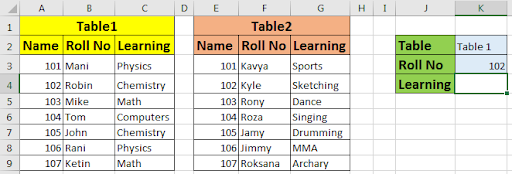Write this formula in K4.

=VLOOKUP(K3,IF(K2="table 1",Table1,Table2),3,0)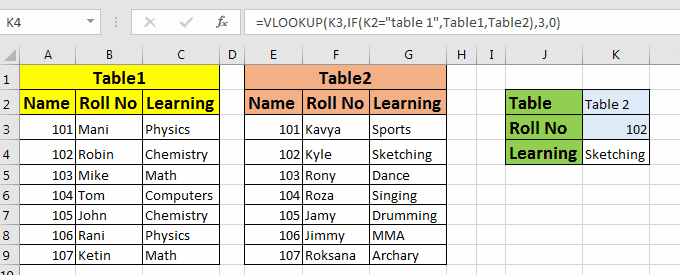How It Works

It is simple. VLOOKUP looks for roll no. in K4. Now at Lookup_Array variable, we placed an IF function that checks value in K2. if K2 contains “Table 1”, table1 is selected, else table2. And then usual VLOOKUP work is done.

Above method works when tables are identical. If they are not, then you may have to put an if condition at col_index_num to get appropriate column. If you have more than 2 table arrays, you can use nested IFs in excel to choose from a number of tables.

Related Articles:

IFERROR and VLOOKUP function

The VLOOKUP Function in Excel

IF, ISNA and VLOOKUP function

VLOOKUP Multiple Values

Popular Articles:

COUNTIFS Two Criteria Match

COUNTIF in Excel 2016

1.